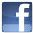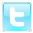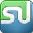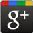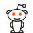# Proof that Bill Gates is the Devil

The real name of "the" Bill Gates is William Henry Gates III. Nowadays he is known as Bill Gates (III), where "III" means the order of third (3rd.)

By converting the letters of his current name to the ASCII-values and adding his (III), you get the following:

B 66
I 73
L 76
L 76
G 71
A 65
T 84
E 69
S 83
+ 3
--------------
666 !!

Some might ask, "How did Bill Gates get so powerful?" Coincidence? Or just the beginning of mankind's ultimate and total enslavement???

Before you decide, consider the following:

M S - D O S 6 . 2 1
77+83+45+68+79+83+32+54+46+50+49 = 666

W I N D O W S 9 5
87+73+78+68+79+87+83+57+53+1 = 666

Coincidence? You decide...